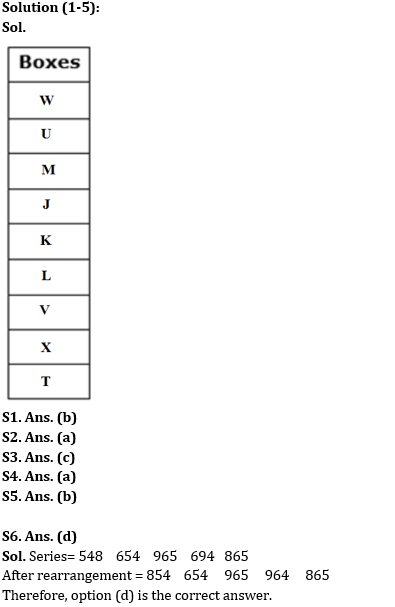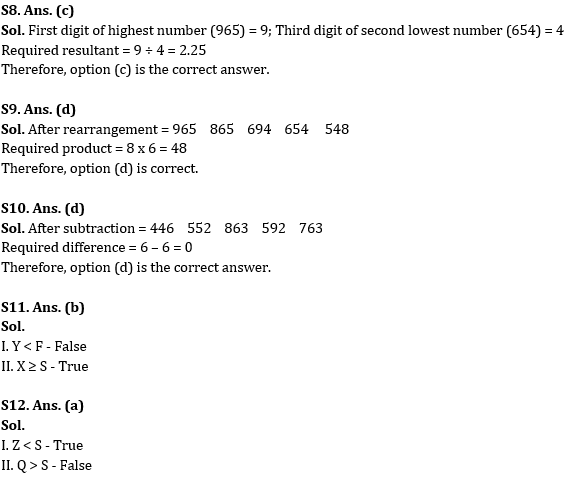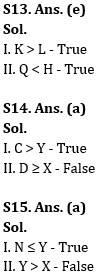Latest Banking jobs   »

# Reasoning Ability Quiz For RBI Grade B/ ECGC PO/ SIDBI Grade A Prelims 2022- 26th May

Directions (1-5): Study the following information carefully and answer the given questions.
Nine boxes i.e., J, K, L, M, T, U, V, W, and X are kept one above the other in a stack. The lowermost stack is numbered 1, the stack above it is numbered 2, and so on until the topmost stack is numbered 9. All the information is not necessarily in the same order.
Three boxes are placed between box J and box X. Two boxes are placed between Box X and Box K. Box W is placed four places above Box K. Four boxes are placed between Box W and Box L. Two boxes are placed between Box L and Box M. Box U is placed adjacent to Box M. Box T is placed below Box V but not immediate below. Box V does not place at the top.

Q1. How many boxes are placed between Box U and Box J?
(a) Three
(b) One
(c) Two
(d) None
(e) More than three

Q2. Which amongst the following box is placed three places below Box L?
(a) Box T
(b) Box X
(c) Box V
(d) Box U
(e) None of these

Q3. What is the position of Box K from top?
(a) Second
(b) Third
(c) Fifth
(d) Seventh
(e) Fourth

Q4. How many boxes are placed below box J?
(a) Five
(b) Three
(c) One
(d) Six
(e) Two

Q5. Which amongst the following box is placed on topmost position?
(a) U
(b) W
(c) J
(d) M
(e) None of these

Directions (6-10): Study the following series carefully and answer the questions accordingly.

548   654   965   694   865

Q6. If all the digits in each number are arranged in descending order within the number then how many numbers will remain the same?
(a) Two
(b) One
(c) More than three
(d) Three
(e) None of these

Q7. If the positions of the first and the third digit of each number are interchanged then what will be the sum of all the digits of the second-lowest number thus formed?
(a) 23
(b) 19
(c) 16
(d) 21
(e) None of these

Q8. What will be the resultant if the first digit of the highest number is divided by the third digit of the second lowest number?
(a) 2.10
(b) 3.20
(c) 2.25
(d) 1.75
(e) None of these

Q9. If all the numbers are arranged in descending order from the left to the right then which of the following will be the product of the first and the second digit of the number which is second from the left end?
(a) 64
(b) 52
(c) 56
(d) 48
(e) None of these

Q10. If one is subtracted from the first digit and two is subtracted from the third digit of each of the numbers then what will be the difference between the second digit of the highest number and third digit of the lowest number thus formed?
(a) 4
(b) 1
(c) 2
(d) 0
(e) None

Directions (11-15): In the following questions assuming the given statement to be true, find which of the following conclusion(s) is/are definitely true, and then give your answers accordingly.

Q11. Statements: Y ≤ D = C, F ≤ X < C, F = S < Q
Conclusions:
I. Y < F
II. X ≥ S
(a) Only I is true
(b) Only II is true
(c) Either I or II is true
(d) Neither I nor II is true
(e) Both I and II are true

Q12. Statements: B = S ≥ N < P, K = Z < B, Q ≥ P
Conclusions:
I. Z < S
II. Q > S
(a) Only I is true
(b) Only II is true
(c) Either I or II is true
(d) Neither I nor II is true
(e) Both I and II are true

Q13. Statements: K > T ≥ Q, Q = L < R, R ≤ U = H
Conclusions:
I. K > L
II. Q < H
(a) Only I is true
(b) Only II is true
(c) Either I or II is true
(d) Neither I nor II is true
(e) Both I and II are true

Q14. Statements: U = C > X, B = V ≤ X, B > Y < D
Conclusions:
I. C > Y
II. D ≥ X
(a) Only I is true
(b) Only II is true
(c) Either I or II is true
(d) Neither I nor II is true
(e) Both I and II are true

Q15. Statements: W < Y ≥ F < X, F = N
Conclusions:
I. N ≤ Y
II. Y > X
(a) Only I is true
(b) Only II is true
(c) Either I or II is true
(d) Neither I nor II is true
(e) Both I and II are true

Solutions#### Congratulations!